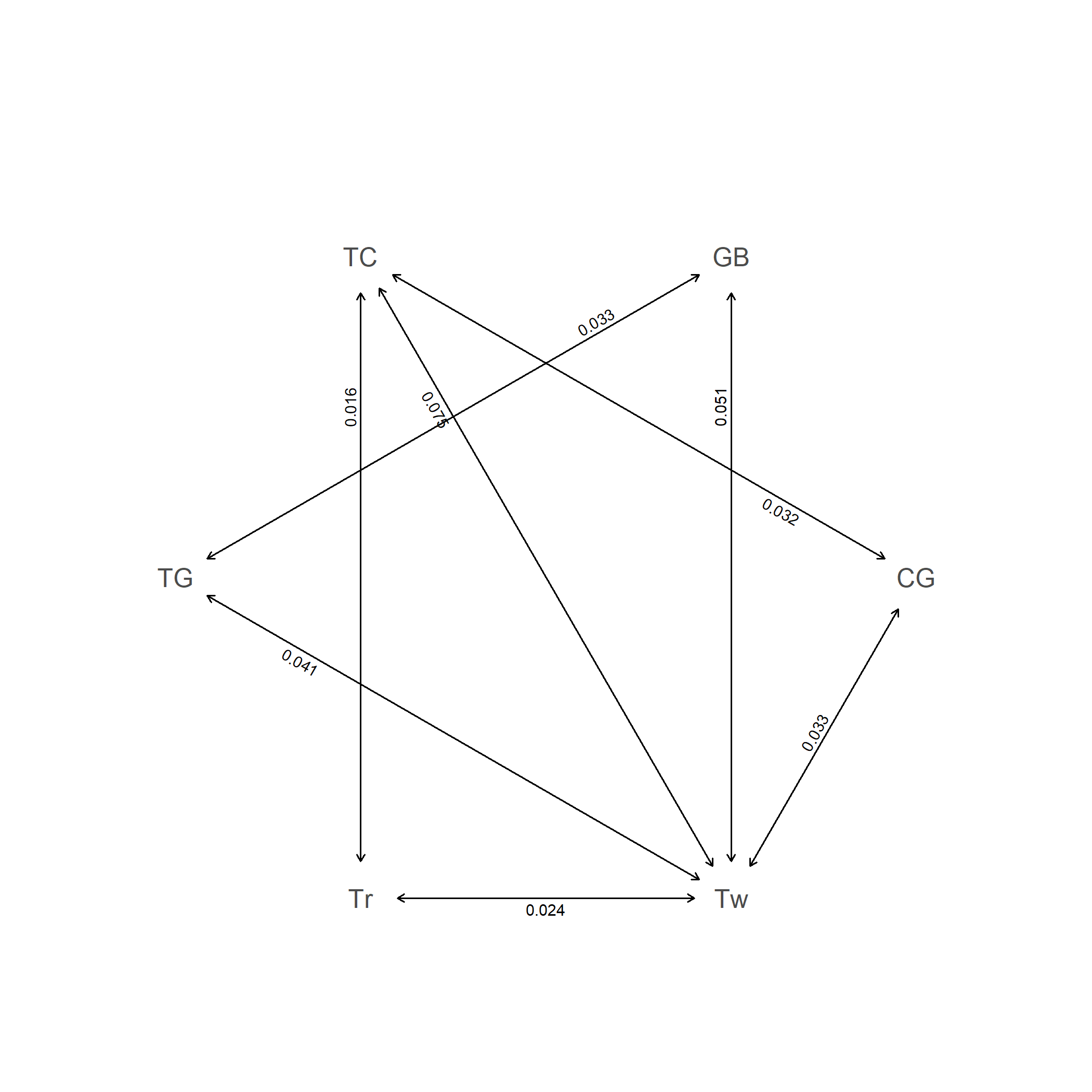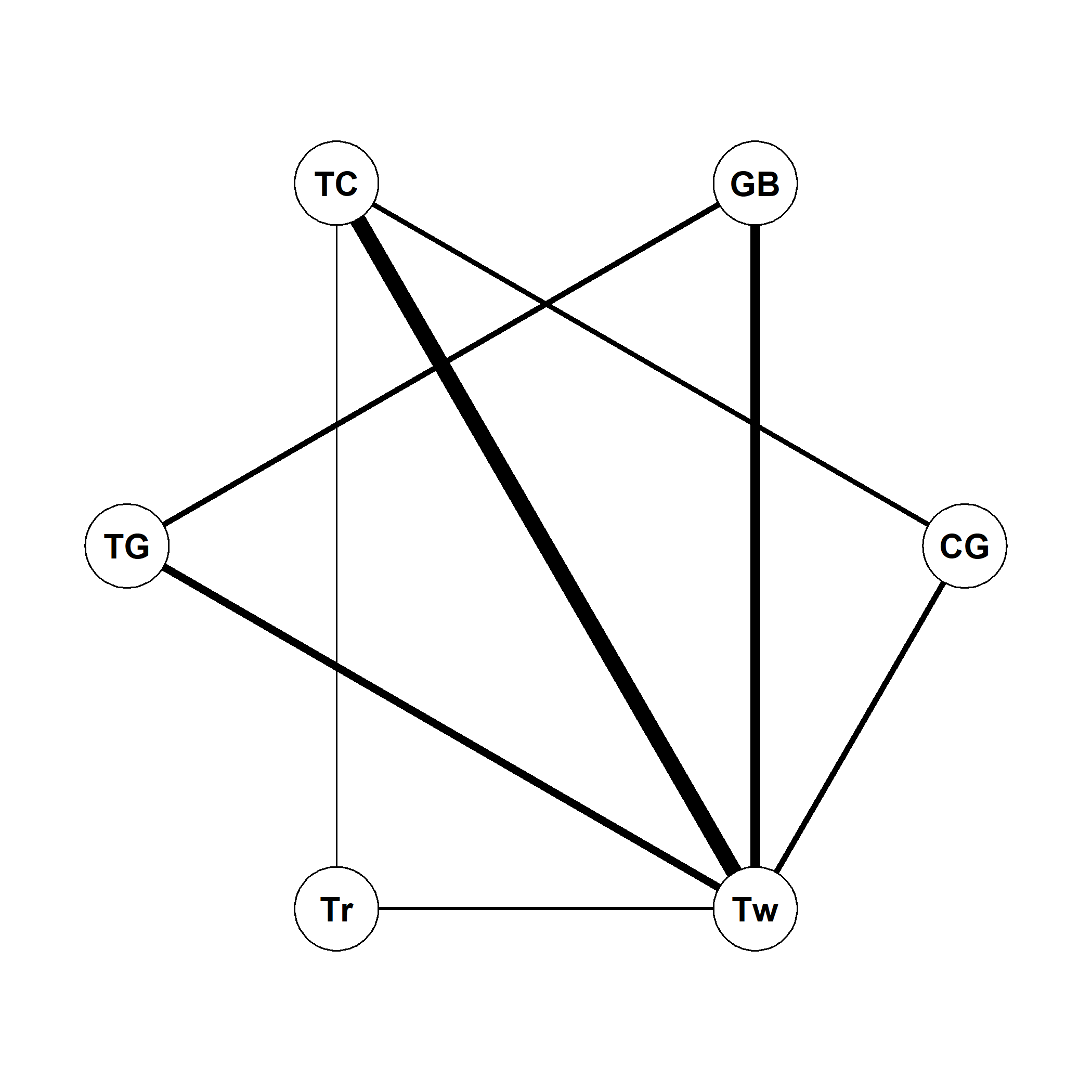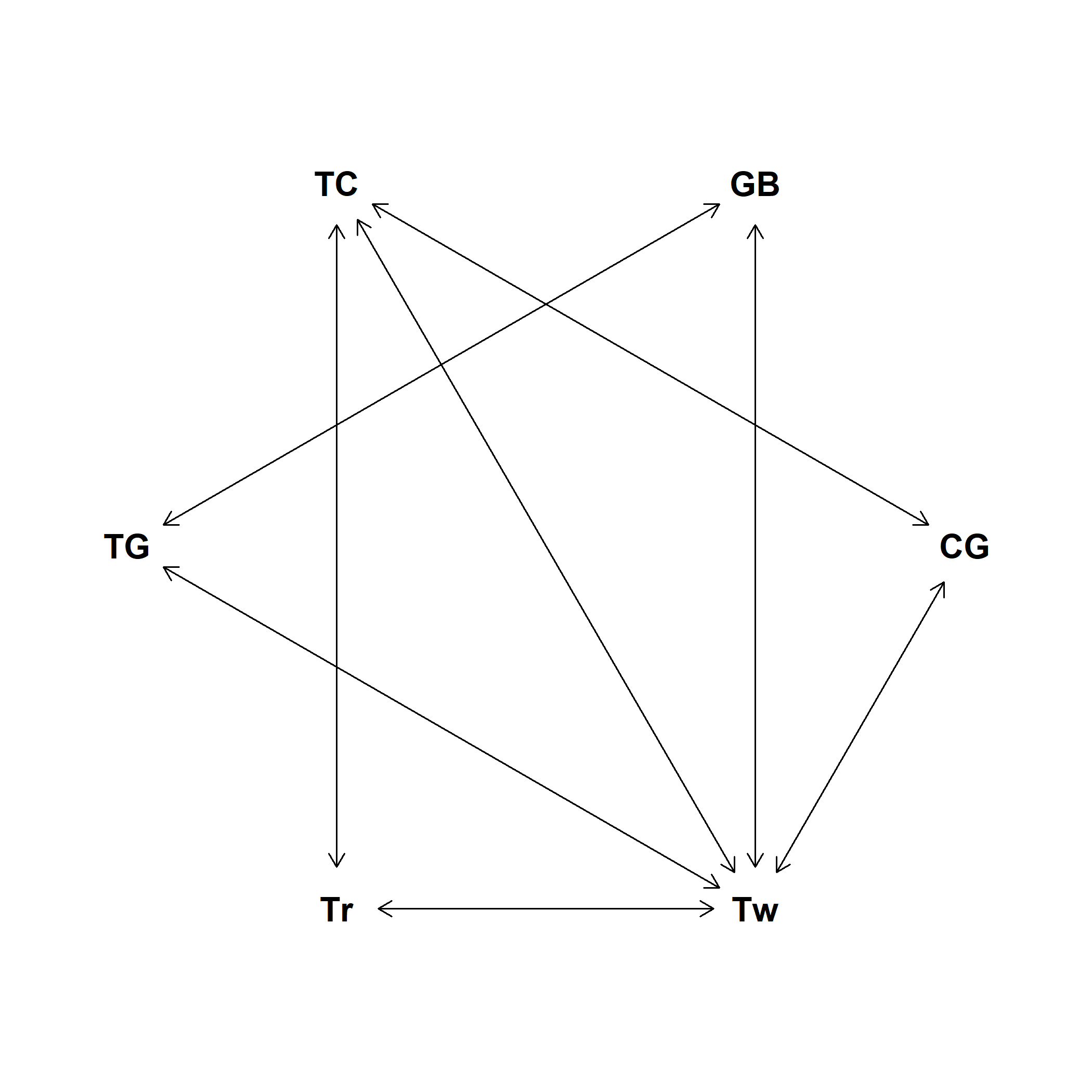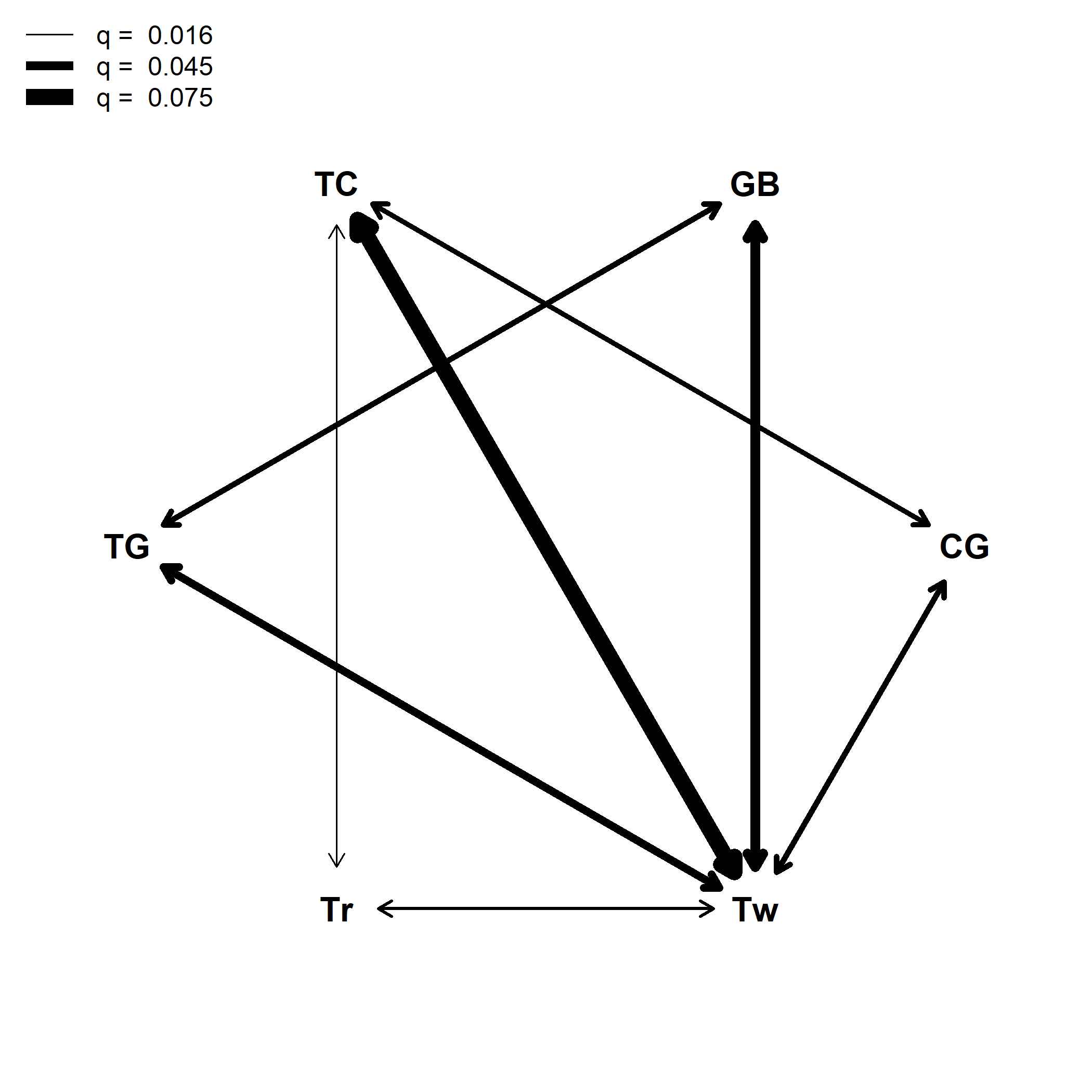## Monday, September 28, 2020

### Graphing a fitted Mk model with different weight arrows for different rates

In response to my post yesterday showing how to create a customized plot of a fitted Mk model with phytools one user asked:

“Very cool! can we scale the arrow width according to the transition rate?”

This is not yet an option in the plot method, per se… but, in R, it's usually not a question of 'if' but 'how'?

Let's see.

Going back to our example from yesterday of the evolution of ecomorph state in Anolis lizards, let's re-load our phylogeny and dataset, as follows:

``````library(phytools)
data(anoletree)
anolis.tree<-as.phylo(anoletree)
ecomorph<-as.factor(getStates(anoletree,"tips"))
``````

Now we can proceed to fit our model. We'll focus on a slightly easier case by imagining that we're fitting a symmetric (`"SYM"`) transition model.

``````fitSYM<-fitMk(anolis.tree,ecomorph,model="SYM",
pi="fitzjohn")
print(fitSYM,digits=4)
``````
``````## Object of class "fitMk".
##
## Fitted (or set) value of Q:
##         CG      GB      TC      TG      Tr      Tw
## CG -0.0652  0.0000  0.0318  0.0000  0.0000  0.0334
## GB  0.0000 -0.0835  0.0000  0.0326  0.0000  0.0508
## TC  0.0318  0.0000 -0.1223  0.0000  0.0156  0.0750
## TG  0.0000  0.0326  0.0000 -0.0736  0.0000  0.0409
## Tr  0.0000  0.0000  0.0156  0.0000 -0.0391  0.0235
## Tw  0.0334  0.0508  0.0750  0.0409  0.0235 -0.2237
##
## Fitted (or set) value of pi:
##     CG     GB     TC     TG     Tr     Tw
## 0.0011 0.0015 0.0079 0.0020 0.0002 0.9874
## due to treating the root prior as (a) nuisance.
##
## Log-likelihood: -72.0732
##
## Optimization method used was "nlminb"
``````

Now, let's plot our model in the ordinary way - however, we'll save the object that's returned invisibly by our plotting method:

``````par(mar=rep(0.1,4))
obj<-plot(fitSYM,show.zeros=FALSE)
``````Now let's look at our object:

``````obj
``````
``````##   states    x             y
## 1     CG  1.0  0.000000e+00
## 2     GB  0.5  8.660254e-01
## 3     TC -0.5  8.660254e-01
## 4     TG -1.0  1.224606e-16
## 5     Tr -0.5 -8.660254e-01
## 6     Tw  0.5 -8.660254e-01
``````

We can see that it consists of the coordinates of all the vertices of our plot.

To start, let's draw lines between each vertex - but this time adjusting for the line with depending on the transition rate. We'll also only draw a line if the transition rate is larger than a particular, small tolerance value - let's say 1e-4.

As such, we can begin by figuring out our desired line width. Let's say that our line width should range from 1 to 10. (Some R graphical devices support non-integer line widths - others don't, so what we see will depend a little bit on which device we use to render our plot!)

``````Qhat<-as.Qmatrix(fitSYM)
Qhat
``````
``````## Estimated Q matrix:
##             CG          GB          TC          TG          Tr          Tw
## CG -0.06524880  0.00000000  0.03181507  0.00000000  0.00000000  0.03343374
## GB  0.00000000 -0.08346690  0.00000000  0.03262396  0.00000000  0.05084294
## TC  0.03181507  0.00000000 -0.12233268  0.00000000  0.01555222  0.07496539
## TG  0.00000000  0.03262396  0.00000000 -0.07356945  0.00000000  0.04094548
## Tr  0.00000000  0.00000000  0.01555222  0.00000000 -0.03909540  0.02354318
## Tw  0.03343374  0.05084294  0.07496539  0.04094548  0.02354318 -0.22373073
``````
``````tol<-1e-4
q.range<-range(Qhat[Qhat>tol])
lwd.range<-c(1,10)
Q.lwd<-matrix(0,nrow(Qhat),ncol(Qhat),
dimnames=dimnames(Qhat))
for(i in 1:nrow(Q.lwd)) for(j in 1:ncol(Q.lwd)){
if(Qhat[i,j]>tol){
Q.lwd[i,j]<-(Qhat[i,j]-q.range)/diff(q.range)*
diff(lwd.range)+lwd.range
}
}
Q.lwd
``````
``````##          CG       GB        TC       TG       Tr        Tw
## CG 0.000000 0.000000  3.463522 0.000000 0.000000  3.708720
## GB 0.000000 0.000000  0.000000 3.586054 0.000000  6.345893
## TC 3.463522 0.000000  0.000000 0.000000 1.000000 10.000000
## TG 0.000000 3.586054  0.000000 0.000000 0.000000  4.846611
## Tr 0.000000 0.000000  1.000000 0.000000 0.000000  2.210484
## Tw 3.708720 6.345893 10.000000 4.846611 2.210484  0.000000
``````

Since our matrix is symmetric (as per our fitted model), we only have to traverse the upper triangular half of our matrix.

Just so the plot doesn't look really dumb, I'm going to use plotrix to overlay circles with our character states at all the vertices of our graph.

``````library(plotrix)
par(mar=rep(0.1,4))
plot(NA,xlim=c(-1.2,1.2),ylim=c(-1.2,1.2),
axes=FALSE,bty="n",xlab="",ylab="")
for(i in 1:nrow(Qhat)){
for(j in i:ncol(Qhat)){
if(Q.lwd[i,j]>0){
segments(obj\$x[i],obj\$y[i],obj\$x[j],obj\$y[j],
lwd=Q.lwd[i,j])
}
}
}
invisible(mapply(draw.circle,obj\$x,obj\$y,col="white",
text(obj\$x,obj\$y,obj\$states,cex=1.3,font=2)
``````OK, that's kind of close. The problem we still have is that our lines are not arrows - and they go all the way to each vertex of the graph, instead of leaving space for our character state labels.

To figure out where to draw the lines so that they end where we want them, we're going to need to use a bit of trigonometry!

Let's do that & try again:

``````par(mar=rep(0.1,4))
plot(NA,xlim=c(-1.2,1.2),ylim=c(-1.2,1.2),
axes=FALSE,bty="n",xlab="",ylab="")
for(i in 1:nrow(Qhat)){
for(j in i:ncol(Qhat)){
if(Q.lwd[i,j]>0){
slope<-abs(diff(obj\$y[c(i,j)])/diff(obj\$x[c(i,j)]))
x0<-obj\$x[i]+0.1*cos(atan(slope))*sign(diff(obj\$x[c(i,j)]))
x1<-obj\$x[j]+0.1*cos(atan(slope))*sign(diff(obj\$x[c(j,i)]))
y0<-obj\$y[i]+0.1*sin(atan(slope))*sign(diff(obj\$y[c(i,j)]))
y1<-obj\$y[j]+0.1*sin(atan(slope))*sign(diff(obj\$y[c(j,i)]))
arrows(x0,y0,x1,y1,length=0.1,code=3)
}
}
}
text(obj\$x,obj\$y,obj\$states,cex=1.3,font=2)
``````Cool. That worked.

Finally, let's combine the two plots:

``````par(mar=rep(0.1,4))
plot(NA,xlim=c(-1.2,1.2),ylim=c(-1.2,1.2),
axes=FALSE,bty="n",xlab="",ylab="")
for(i in 1:nrow(Qhat)){
for(j in i:ncol(Qhat)){
if(Q.lwd[i,j]>0){
slope<-abs(diff(obj\$y[c(i,j)])/diff(obj\$x[c(i,j)]))
x0<-obj\$x[i]+0.1*cos(atan(slope))*sign(diff(obj\$x[c(i,j)]))
x1<-obj\$x[j]+0.1*cos(atan(slope))*sign(diff(obj\$x[c(j,i)]))
y0<-obj\$y[i]+0.1*sin(atan(slope))*sign(diff(obj\$y[c(i,j)]))
y1<-obj\$y[j]+0.1*sin(atan(slope))*sign(diff(obj\$y[c(j,i)]))
arrows(x0,y0,x1,y1,length=0.1,code=3,lwd=Q.lwd[i,j])
}
}
}
text(obj\$x,obj\$y,obj\$states,cex=1.3,font=2)
par(lend=1)
legend("topleft",
c(paste("q = ",round(q.range,3)),
paste("q = ",round(mean(q.range),3)),
paste("q = ",round(q.range,3))),
lwd=c(lwd.range,mean(lwd.range),lwd.range),
bty="n")
``````That's OK. Phew!

Alright, for non-symmetric transition models I think it would be easier to program it into the `plot.Qmatrix` function…. Look for that in a future phytools update!